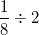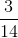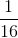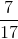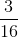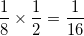# SSAT Middle Level Math : How to divide fractions

## Example Questions

### Example Question #31 : Fractions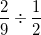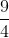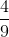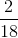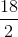Explanation:To divide fractions, we multiply by the reciprocal. In order to find the reciprocal, we simply flip the fraction over. The numerator becomes the denominator and the denominator becomes the numerator.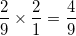### Example Question #32 : Fractions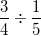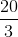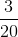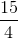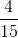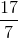Explanation:To divide fractions, we multiply by the reciprocal. In order to find the reciprocal, we simply flip the fraction over. The numerator becomes the denominator and the denominator becomes the numerator.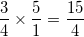### Example Question #171 : Operations With Fractions And Whole Numbers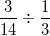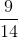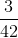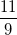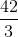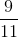Explanation:To divide fractions, we multiply by the reciprocal. In order to find the reciprocal, we simply flip the fraction over. The numerator becomes the denominator and the denominator becomes the numerator.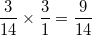### Example Question #531 : Number & Operations With Fractions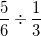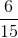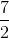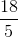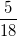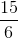Explanation:To divide fractions, we multiply by the reciprocal. In order to find the reciprocal, we simply flip the fraction over. The numerator becomes the denominator and the denominator becomes the numerator.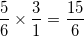### Example Question #401 : Fractions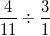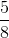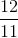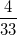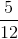Explanation:To divide fractions, we multiply by the reciprocal. In order to find the reciprocal, we simply flip the fraction over. The numerator becomes the denominator and the denominator becomes the numerator.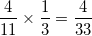### Example Question #403 : Fractions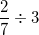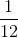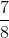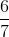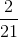Explanation:To divide fractions, we multiply by the reciprocal. In order to find the reciprocal, we simply flip the fraction over. The numerator becomes the denominator and the denominator becomes the numerator.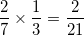### Example Question #404 : Fractions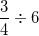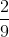Explanation:To divide fractions, we multiply by the reciprocal. In order to find the reciprocal, we simply flip the fraction over. The numerator becomes the denominator and the denominator becomes the numerator.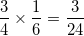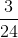can be reduced by dividing both sides by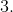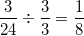### Example Question #405 : Fractions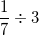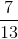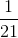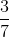Explanation:To divide fractions, we multiply by the reciprocal. In order to find the reciprocal, we simply flip the fraction over. The numerator becomes the denominator and the denominator becomes the numerator.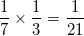### Example Question #406 : Fractions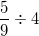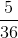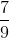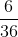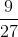Explanation:To divide fractions, we multiply by the reciprocal. In order to find the reciprocal, we simply flip the fraction over. The numerator becomes the denominator and the denominator becomes the numerator.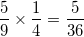### Example Question #407 : Fractions